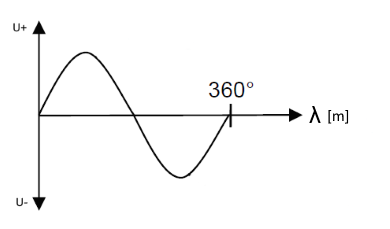# Frequency and wavelengths

Online calculation and formulas for calculating frequency and wavelengths

## Calculate frequency and wavelengths

On this page you can calculate the wavelengths to a certain frequency, or the frequency to a wavelength. Wavelengths for electrical oscillations, light and sound can be calculated.

Frequency or wavelength calculator

 What should be calculated? Wavelength Frequency Enter the value and select the medium Electric waves in space Electric waves in cables Light Sound waves in the air +20°C Sound waves in the water Frequency Hz kHz MHz GHz THz Wavelength pm nm µm mm m km Decimal places 0 1 2 3 4 6 8 10 Result Frequency Wavelength

Tip: You will find a calculator for calculating frequency and period time here

### Formulas for frequency and wavelength

The wavelength is the length of one period of an oscillation.The wavelength depends on the frequency and the speed of propagation of the waves. The following table shows the speed of propagation of different waves in different media, as used in the calculator above.

Electrical oscillation in free space
v = 300000 km/s
Electrical vibration in cables
v ≈ 240000 km/s
Light waves
v = 300000 km/s
Sound waves in air +20°C
v = 343 m/s
Sound waves in water
v = 1470 m/s

The wavelength $$\displaystyle λ$$ in meters is calculated by taking the propagation speed $$\displaystyle c$$ divided by the frequency $$\displaystyle f$$ teilt.

$$\displaystyle λ= \frac{c}{f}$$

This results in the following formula for calculating the frequency:

$$\displaystyle f= \frac{c}{λ}$$

#### Legend

 $$\displaystyle λ$$ Wavelength in meters $$\displaystyle f$$ Frequency in Hertz $$\displaystyle c$$ Propagation speed in meters / second